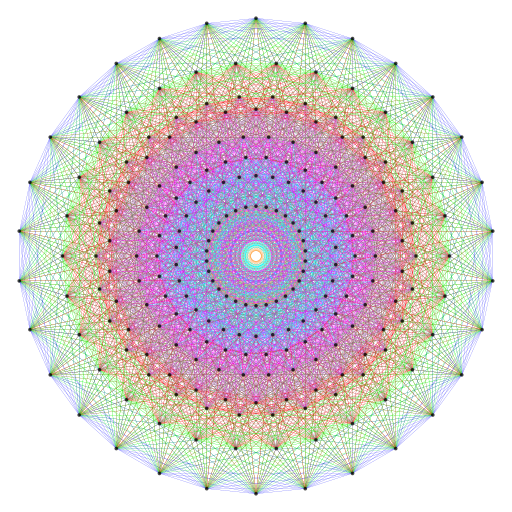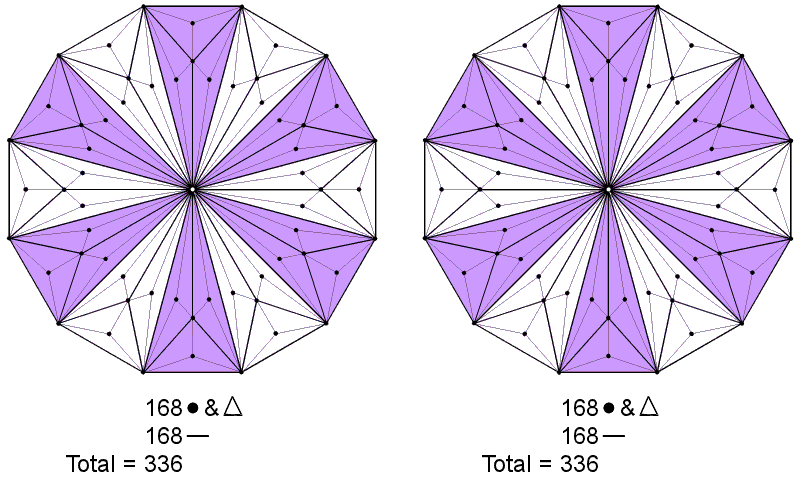Home

 << Previous    1...   15  16    18  19  ...24    Next >>

17. The pair of Type C dodecagons embodies the number of edges of the 421 polytopeEach half of the 421 polytope has 3360 edges. Constructed from Type B triangles, each of the two Type C dodecagons has 336 corners, sides & triangles surrounding its centre.

A Type C polygon has Type B triangles as its sectors. Apart from their shared centre, there are five corners, 14 sides & nine triangles per sector, i.e., 28 geometrical elements. Surrounding the centre of the Type C dodecagon are (12×28=336) geometrical elements. They comprise [12×(5+9)=168] corners & triangles and (12×14=168) sides. This 168:168 division is characteristic of holistic systems displaying this parameter, e.g., the 336 yods lining the 126 sides of the 42 triangles surrounding the centre of the 3-dimensional Sri Yantra consist of 168 yods on the sides of the 21 triangles in each half of it. (336+336=672) geometrical elements surround the centres of the two separate Type C dodecagons as 336 sides and 336 corners & triangles. This property is the counterpart of the 672 yods intrinsic to each set of seven enfolded Type B polygons and surrounding their centres that was discussed in #12. It is also the counterpart of other sacred geometries previously analysed. However, unlike yods, the number 10 cannot be meaningfully assigned to lines and triangles. Instead, we must remember that — as considered earlier for the Type B polygons — 10 overlapping Trees of Life can represent the single Tree composed of 10 Sephiroth. Surrounding the centres of the 20 separate, Type C dodecagons that are part of their inner form are 6720 geometrical elements (3360 sides, 3360 corners & triangles). As a holistic system in itself, the pair of Type C dodecagons through the 10-fold Tree of Life generates the number 6720. This is the number of edges in the 421 polytope whose vertices determine the roots of E8, which is the exceptional Lie group that describes the unified symmetry of superstrings whose 10 dimensions are mapped by these 10 Trees of Life.

The 12th dodecagonal number is 672 (see Table 2 here). This is the number of geometrical elements surrounding the centres of the two Type C dodecagons.

 << Previous    1...   15  16    18  19  ...24    Next >>

Home